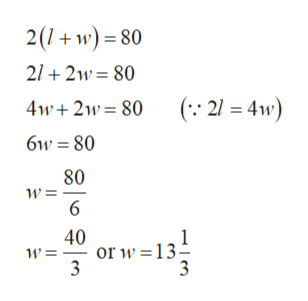# Twice the length of a rectangle is equal to four times its width. The perimeter of a rectangle is 80 inches. Find the dimensions of the rectangle.

Question
28 views

Twice the length of a rectangle is equal to four times its width. The perimeter of a rectangle is 80 inches. Find the dimensions of the rectangle.

check_circle

Step 1

Let l and w be the length and width of the rectangle, respectively.

Given that, twice the length of a rectangle is equal to four times its width. That is,

Step 2

Given that, the perimeter of a rect...help_outlineImage Transcriptionclose2w)80 2/ 2w 80 ( 21 4w) 4w 2w 80 6w 80 80 w 6 40 or w=13 3 3 fullscreen

### Want to see the full answer?

See Solution

#### Want to see this answer and more?

Solutions are written by subject experts who are available 24/7. Questions are typically answered within 1 hour.*

See Solution
*Response times may vary by subject and question.
Tagged in

### Algebra